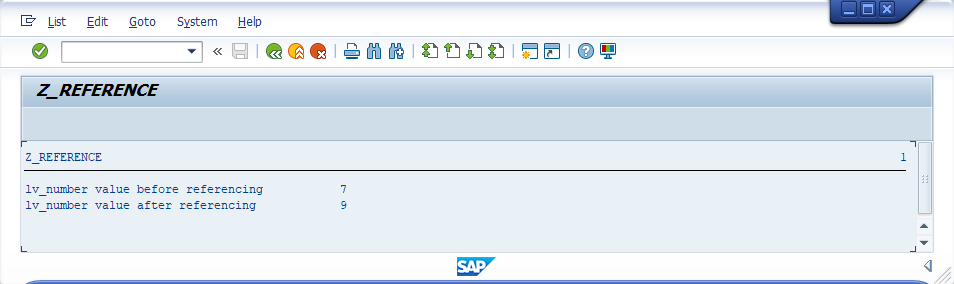###### Summary -

In this topic, we described about the below sections -

Reference variable contain references (i.e. pointing to the memory location of the another variable). ABAP supports data references and object references.

The actual contents of the reference variable are not visible in the ABAP program. The contents of the reference variable are the address of memory location.

Reference variables can be handled as other data objects that are defined as an elementary data type. Reference variable can be defined as a complex data object component such as a structure or internal table.

#### Syntax -

``DATA {reference-variable} TYPE REF TO {data-type} VALUE IS INITIAL.``

REF TO - Declares a reference variable.

There are two types of reference variables and those are -

• Data reference variables
• Object reference variables

The syntax for creating data type of data reference variable is -

``````TYPES t_dref TYPE REF TO DATA.
``````

The syntax for creating a data reference variable either by referring to the above data type or using -

``````DATA dref TYPE REF TO DATA.
``````

Reference variables are initialized when they declared. They do not point to an object. We cannot dereference an initialized reference variable.

A data reference variable can points to a data object when -

• Creating a data object dynamically.
• Getting a reference to a data object.
• Assigning an existing data reference to it from another data reference variable.

We can assign references to data reference variables using the MOVE statement or the assignment operator (=), and also when an internal table initialized or pass parameters to a procedure.

We cannot assign to object reference variables or other variables. After the assignment, the reference in the target variable points to the same data object in the source variable.

#### Example -

Write a simple program to get clear understanding of reference variable.

#### Code -

``````*&---------------------------------------------------------------------*
*& Report  Z_REFERENCE
*&---------------------------------------------------------------------*
*& Program Written by TUTORIALSCAMPUS
*&---------------------------------------------------------------------*

REPORT  Z_REFERENCE.

* local variable lv_number declaration
DATA: lv_number TYPE I VALUE 7.

* local reference variable lr_number declaration
DATA: lr_number TYPE REF TO I.

* Displaying the local variable number before referencing
WRITE : 'lv_number value before referencing ', lv_number.

* Referencing lr_number to lv_number
GET REFERENCE OF lv_number INTO lr_number.

* Changing the value of lv_number using the reference variable lr_number
lr_number->* = 9.

* Displaying the local variable number after referencing & value change
WRITE : /'lv_number value after referencing  ', lv_number.``````

#### Output -#### Explaining Example -

In the above example, each and every statement is preceeded with a comment to explain about the statement. Go through them to get clear picture of example code.

Coming to output, lv_number variable is declared as a local variable and initialized with 7. lr_number is reference variable declared and the reference of lv_number assigned to lr_number using the statement "GET REFERENCE OF".

Now, the lr_number is pointing to the memory location of lv_number where the value of 7 stored. Assigning 9 to the memory area using lr_number indirectly changes the lv_number value. So 7 got replaced with 9 and final output is stored in lv_number.